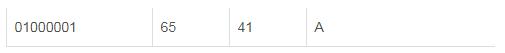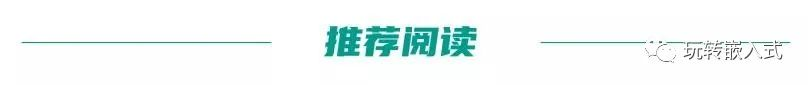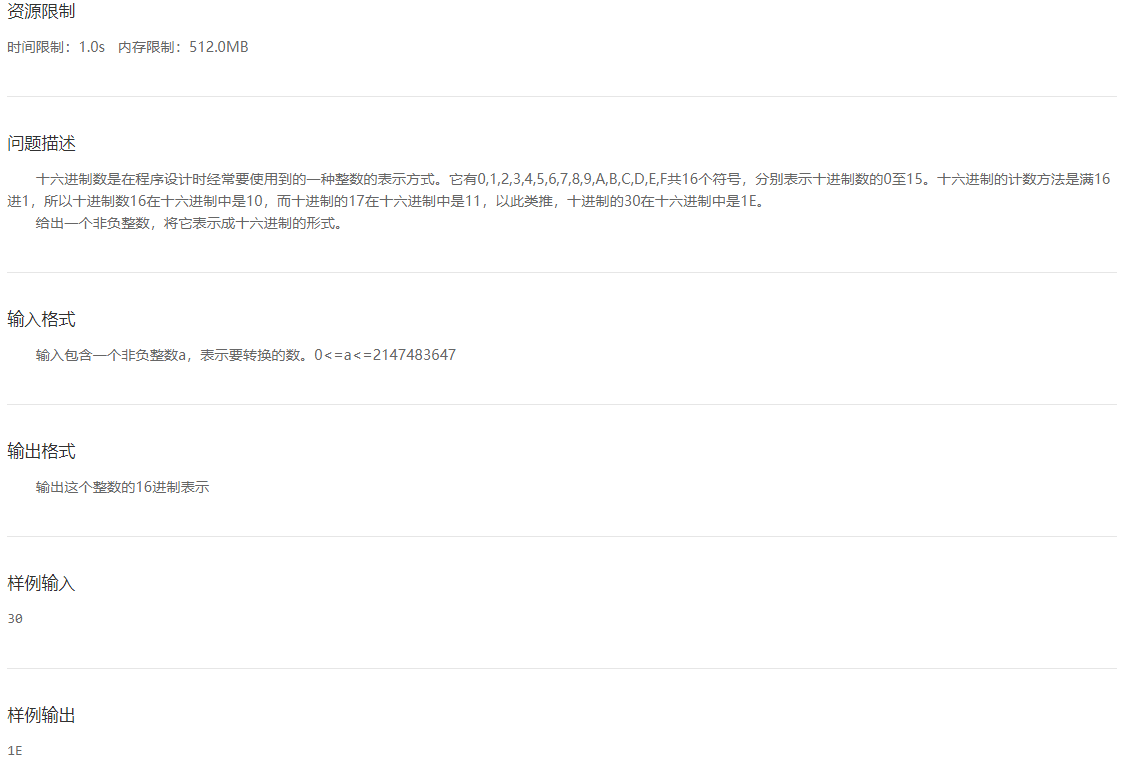• #include<stdio.h>//标准的输入输出流 #include<stdlib.h>//system(“pause”)要用 #include<math.h>//因为后面会有幂次方函数pow（），第一个...//十进制转换为十六进制 int main(void) { int a = 0
#include<stdio.h>//标准的输入输出流
#include<stdlib.h>//system(“pause”)要用
#include<math.h>//因为后面会有幂次方函数pow（），第一个参数是底数，第二个参数是指数
int binary(int m);//十进制转换为二进制
int bajinzhi(int m);//十进制转换为八进制
int shiliu(int m);//十进制转换为十六进制
int main(void)
{
int a = 0; int b, c = 0;
printf(“请输入一个十进制数\n”);//提示用户输入信息
scanf_s("%d", &a);//将你刚刚输进去的变量读入
getchar();
printf(“请输入一个数，如果是想要转换为二进制的就输入1，如果是想要转换为八进制的就输入2，如果是想要转换为16进制的就输入3\n”);
scanf_s("%d", &b);
switch (b)//根据b的数值的不同进入switch case里面的不同分支里面，来转换为用户想要的进制
{//注意每一个case里面都需要有一个break语句，
//如果default在最后一个，那么可以要break，
//也可以不要break，通常为了规范，还是要在每一个语句的下面都添加一个break语句
case 1:
c = binary(a);//将用户输的十进制整数传入，
//该函数的返回值是一个跟用户输入的
//十进制整数相对应的二进制数
printf(“转换为二进制的数为%d\n”, c);
break;
case 2:
printf(“转换为八进制的数为”);
//将用户输的十进制整数传入，
//该函数的返回值是一个跟用户输入的
//十进制整数相对应的八进制数
c = bajinzhi(a);
printf("%d\n", c);
break;
case 3:
printf(“转换为十六进制的数为”);
//将用户输的十进制整数传入，
//该函数的返回值是一个跟用户输入的
//十进制整数相对应的十六进制数
c = shiliu(a);
printf("%d\n", c);
break;
default:
printf(“输入错误，请重新输入\n”);
//如果用户不是输入上面的那些整数的话，
//就代表用户已经输入错误，需要提示用户重新输入
break;
}
system(“pause”);将黑色窗口停留，用户按任意键后才会退出
return 0;//程序正常运行要返回一个0
}
int binary(int m)
{
int i = 0;
int a;
int sum = 0;
while (m)//当m不为0循环就一直进行下去，知道m=0，然后退出循环
{
a = m % 2;
m = m / 2;//十进制数转换为二进制数的具体实现
sum += a * pow(10, i++);
}
return sum;//将你输入的十进制数所对应的二进制数返回
}
int bajinzhi(int m)
{
int i = 0;
int a;
int sum = 0;//为什么要初始化sum等于0，因为sum是用来计算求和的一个变量，所以要初始化为0
while (m)
{
a = m % 8;
m = m / 8;//十进制数转换为八进制数的具体实现
sum += a * pow(10, i++);
}
return sum;//将你输入的十进制数所对应的八进制数返回
}
int shiliu(int m)
{
int i = 0;
int a;
int sum = 0;
while (m)
{
a = m % 16; //十进制数转换为十六进制数的具体实现
m = m / 16;
sum += a * pow(10, i++);
}
return sum;//将你输入的十进制数所对应的十六进制数返回
}


展开全文• 编程时经常用到进制转换、字符转换。比如软件界面输入的数字字符串，如何将字符串...01字符串转十六进制代码实现：voidStrToHex(char*pbDest,char*pbSrc,intnLen){charh1,h2;chars1,s2;inti;for(i=0;i{h1=pbSrc[2*i...编程时经常用到进制转换、字符转换。比如软件界面输入的数字字符串，如何将字符串处理成数字呢？和大家分享一下。01字符串转十六进制代码实现：void StrToHex(char *pbDest, char *pbSrc, int nLen){  char h1,h2;  char s1,s2;  int i;    for (i=0; i    {        h1 = pbSrc[2*i];        h2 = pbSrc[2*i+1];        s1 = toupper(h1) - 0x30; //toupper 转换为大写字母        if (s1 > 9)            s1 -= 7;        s2 = toupper(h2) - 0x30;        if (s2 > 9)            s2 -= 7;        pbDest[i] = s1*16 + s2;    }}02十六进制转字符串代码实现：void HexToStr(char *pszDest, char *pbSrc, int nLen){    char    ddl, ddh;    for (int i = 0; i     {        ddh = 48 + pbSrc[i] / 16;        ddl = 48 + pbSrc[i] % 16;        if (ddh > 57) ddh = ddh + 7;        if (ddl > 57) ddl = ddl + 7;        pszDest[i * 2] = ddh;        pszDest[i * 2 + 1] = ddl;    }    pszDest[nLen * 2] = '\0';}或者：u16 Hex2StringArray (u8 *pSrc,  u16 SrcLen, u8 *pObj){    u16 i=0;    for(i=0;    i    {        sprintf((char *)(pObj + i * 2), "%02X", *(pSrc + i));    }    *(pObj + i * 2) = '\0';    return  (i * 2);}效果：十六进制：0x13 0xAA 0x02转为字符串：13AAA203字符串转十进制代码实现：第一种，如果带负号 这个就是atoi函数的实现：int my_atoi(const char *str){    int value = 0;    int flag = 1; //判断符号    while (*str == ' ')  //跳过字符串前面的空格    {        str++;    }    if (*str == '-')  //第一个字符若是‘-’，说明可能是负数    {        flag = 0;        str++;    }    else if (*str == '+') //第一个字符若是‘+’，说明可能是正数    {        flag = 1;        str++;    }//第一个字符若不是‘+’‘-’也不是数字字符，直接返回0    else if (*str >= '9' || *str <= '0')     {        return 0;        }    //当遇到非数字字符或遇到‘\0’时，结束转化    while (*str != '\0' && *str <= '9' && *str >= '0')    {        value = value * 10 + *str - '0'; //将数字字符转为对应的整形数        str++;    }    if (flag == 0) //负数的情况    {        value = -value;    }    return value;}效果：字符串：-123 转为 -123第二种，如果不带负号void StrtoDec(uint32_t *pbDest, char *pbSrc, int nLen){    int i;    int tmp=0;    if(nLen > 10)        *pbDest = 0;    tmp = 1;    *pbDest = 0;    for (i=nLen-1; i>=0; i--)    {        *pbDest += tmp*(*(pbSrc+i)-'0');        tmp = tmp*10;    }}效果：字符串：123转为 123第三种：包含转为浮点数//m^n函数//返回值:m^n次方.u32 NMEA_Pow(u8 m,u8 n){    u32 result=1;        while(n--)result*=m;        return result;}//str转换为数字,以','或者'*'结束//buf:数字存储区//dx:小数点位数,返回给调用函数//返回值:转换后的数值int NMEA_Str2num(u8 *buf,u8*dx){    u8 *p=buf;    u32 ires=0,fres=0;    u8 ilen=0,flen=0,i;    u8 mask=0;    int res;    while(1) //得到整数和小数的长度    {        if(*p=='-'){mask|=0X02;p++;}//是负数        if(*p==','||(*p=='*'))break;//遇到结束了        if(*p=='.'){mask|=0X01;p++;}//遇到小数点了        else if(*p>'9'||(*p<'0'))   //有非法字符        {               ilen=0;            flen=0;            break;        }           if(mask&0X01)flen++;        else ilen++;        p++;    }    if(mask&0X02)buf++; //去掉负号    for(i=0;i    {          ires+=NMEA_Pow(10,ilen-1-i)*(buf[i]-'0');    }    if(flen>5)flen=5;   //最多取5位小数    *dx=flen;           //小数点位数    for(i=0;i    {          fres+=NMEA_Pow(10,flen-1-i)*(buf[ilen+1+i]-'0');    }     res=ires*NMEA_Pow(10,flen)+fres;    if(mask&0X02)res=-res;             return res;} 效果：字符串：”123.456” 先转为 123456，然后除以1000得到123.45604十进制转字符串代码实现：如果只是单个十进制转字符串，使用sprintf函数就可以了。如果是十进制数组：u16 DectoStr (u8 *pSrc, u16 SrcLen, u8 *pObj){    u16 i=0;    for(i=0;    i    {        sprintf((char *)(pObj + i * 2), "%02d", *(pSrc + i));    }    *(pObj + i * 2) = '\0';    return  (i * 2);}效果：十进制数组13 14转为字符串“1314”05u8、u32转换举个栗子：ASCII码里这里写图片描述 字符‘A’ ， 一个字节8bit ，即u8 十六进制为 0x41 二进制为 0100 0001而对应的十进制为 65 整型65，4个字节32bit，即u32 十六进制为 0x41 二进制为 0000 0000 0000 0000 0000 0000 0100 0001将u32数转换成u8数组注意：这里是字符数组，不是字符串字符串是以空字符(\0)结尾的char数组void U32ToU8Array(uint8_t *buf, uint32_t u32Value){    buf = ((u32Value >> 24) & 0xFF);    buf = ((u32Value >> 16) & 0xFF);    buf = ((u32Value >> 8) & 0xFF);    buf = (u32Value & 0xFF);}效果：整型 50 转字符数组 {‘\0’,’\0’,’\0’,’2’}u8数组转u32void U8ArrayToU32(uint8_t *buf, uint32_t *u32Value){    *u32Value = (buf <<24) + (buf <<16) + (buf <<8) + (buf <<0);}效果：字符数组 {‘\0’,’\0’,’\0’,’2’}转为整型 5006大小端(高低位)转换STM32 默认是小端模式的，那么该如何转为大端？//为大端：pPack = (u8)((len >> 8) & 0xFF);pPack = (u8)(len & 0xFF);//为小端：pPack = (u8)(len & 0xFF);pPack =  (u8)((len >> 8) & 0xFF);效果：len为数据类型为 u16(short)，比如 0x11 0x22，转为u8(usigned char)数组。大端为：pPack (0x11 ) pPack (0x22) 小端为：pPack (0x22) pPack (0x11)【1】C语言，动态展示经典排序算法【2】单片机常用的14个C语言算法，看过的都成了大神！【3】插入排序：最直观的排序算法【4】冒泡排序，经典的排序算法【5】卡尔曼滤波算法，啥原理？【6】C语言编程建议和技巧，同样适用于单片机的学习展开全文• 在写好字符转换函数dtoc()的前提下，想到，给定值可以以16为界分为两部分，因此需要判断num < 16? 如果小于16，可直接打印结果；大于等于16，则需要求余求商，此时判断条件变为：商是否大于等于16（另一种说法：...
题目：思路：求余、求商，倒序排放，switch语句
在写好字符转换函数dtoc()的前提下，想到，给定值可以以16为界分为两部分，因此需要判断num < 16? 如果小于16，可直接打印结果；大于等于16，则需要求余求商，此时判断条件变为：商是否大于等于16（另一种说法：是否大于15），如果大于15，则需要一直求商，直至商小于16，在此期间，将所有的商（转换后的商）依次放到定义的字符数组。最后将商转换后放到数组中。循环打印数组中字符。
代码：
运行环境：Code::Blocks 20.03
#include <stdio.h>

//该函数时为了将1-15转换为字符；
char  dtoc(int a)
{
char i;
switch(a)
{
case 0: i = '0';
break;
case 1: i = '1';
break;
case 2: i = '2';
break;
case 3: i = '3';
break;
case 4: i = '4';
break;
case 5: i = '5';
break;
case 6: i = '6';
break;
case 7: i = '7';
break;
case 8: i = '8';
break;
case 9: i = '9';
break;
case 10: i = 'A';
break;
case 11: i = 'B';
break;
case 12: i = 'C';
break;
case 13: i = 'D';
break;
case 14: i = 'E';
break;
default: i = 'F';
break;
}

return i;
}

int main(void)
{
int i, j;
unsigned long int num;
char hs;

scanf("%d", &num);

if(num <16) //判断num是否大于等于16，如果小于16，可直接打印出结果；
{
hs = dtoc(num);
printf("%c", hs);

return 0;

}

while(num > 15) //如果给定值大于15，则求余，并放到数组hs[]中，且求出商；循环计算，直到商小于等于15
hs[i] = dtoc(num%16);
num = num / 16;
i++;
}

hs[i] = dtoc(num);//将最后的商放到数组hs[]中；

for(j = i; j >= 0; j--) //循环打印hs[]中的元素，注意：hs[]中元素为逆序排放
{
printf("%c", hs[j]);
}

return 0;
}

                              /*菜鸟一枚，请多指教*/
End



展开全文• c语言写一个函数十进制转换成十六进制 网上找到一些，感觉有占乱
• C语言十进制转换为十六进制的方法有很多，可以使用for循环和while循环，也可以使用函数。下面我们就来看看具体的方法介绍。十进制数具有基数10并且包括以下数字的数字： 0 1 2 3 4 5 6 7 8 9十六进制数具有基数16...
C语言中十进制转换为十六进制的方法有很多，可以使用for循环和while循环，也可以使用函数。下面我们就来看看具体的方法介绍。十进制数具有基数10并且包括以下数字的数字：  0 1 2 3 4 5 6 7 8 9十六进制数具有基数16并包括以下值：  0 1 2 3 4 5 6 7 8 9 A B C D E F，其中，A = 10，B = 11，C = 12，D = 13，E = 14，F = 15。C语言中十进制转换成十六进制的代码如下while循环：#includeint main() {long int decimalNumber,remainder,quotient;int i=1,j,temp;char hexadecimalNumber;printf("输入十进制数任意: ");scanf("%ld",&decimalNumber);quotient = decimalNumber;while(quotient!=0) {temp = quotient % 16;if( temp < 10)temp =temp + 48; elsetemp = temp + 55;hexadecimalNumber[i++]= temp;quotient = quotient / 16;}printf("十进制数的等效十六进制值 %d: ",decimalNumber);for (j = i -1 ;j> 0;j--)printf("%c",hexadecimalNumber[j]);return 0;}for循环#include#includeint main(){int decimal_number, remainder, hexadecimal_number = 0;int count;printf("Enter a Decimal Number:\t");scanf("%d", &decimal_number);for(count = 0; decimal_number > 0; count++){remainder = decimal_number % 16;hexadecimal_number = hexadecimal_number + remainder * pow(10, count);decimal_number = decimal_number / 16;}printf("\nHexadecimal Equivalent:\t%d\n", hexadecimal_number);return 0;}使用函数#include#includeint decimal_to_hexadecimal(int x){int hexadecimal_number, remainder, count = 0;for(count = 0; x > 0; count++){remainder = x % 16;hexadecimal_number = hexadecimal_number + remainder * pow(10, count);x = x / 16;}return hexadecimal_number;}int main(){int decimal_number, result;printf("Enter a Decimal Number:\t");scanf("%d", &decimal_number);result = decimal_to_hexadecimal(decimal_number);printf("\nHexadecimal Equivalent:\t%d\n", result);return 0;}
展开全文• C语言——十进制转为二进制、八进制、十六进制函数转换 /*** * Copyright(C) 2011, SKYCNC * All rights reserved * * 程序名称：dec_to_bin_oct_hex * 功 能：输入一个十进制数，将其转化为二进制、...
• ﻿﻿ int convert(int a,char *p)//a为字符串长度，p为需要转换字符串指针 {   int i,j,sum;    sum=0;  for(i=0;i  {   if(*(p+i)='a')   j=(int)(*(p+i))-87;   else if(*(p+i)='A')  ...
• ##不甘平凡#最近在学习python，不过跟着课本的作业题目：分别就计算二进制110110011、八进制256和十六进制的数字a4b5，并转化为十进制求和。不过写过程中遇到了个问题：如何在python中输入二进制数字？在网上百度了...
• /*********十进制转为十六进制函数******** 第一个参数为要被转换的十进制， 第二个为转换完成保存的十六进制的位置， 第三个参数为转换后十六进制的长度。 *******************/ void To_Hex(unsi
• PAGE PAGE #/ 4 实验课题一将一个十进制数转换成另外一个 P 进制数字符串(可以是二 进制到十六进制) 转换函数的原 型为 void Convert (int n,char str[], unsigned P; n输入待转换的数 str :输出转换好的P进制字符串...
• C语言规定，“%x"，和”%d“分别代表十六进制十进制数的输入和输出格式控制符。  过程实现：1.创建一个C文件。  2.利用格式数入函数scanf(),从键盘中获取一个十进制整数，利用格式输出函数将转换后的结果输出。 ...
• 关于十进制转十六进制 十进制转十六进制的方法网上介绍的很多，这里简单说明一下。用十进制的数除以十六，得到的余数保留，得到的商继续除以十六，直至除到商为0为止，将先得到的余数排在最右边，后得到的按照顺序...
• C语言某个程序当中需要把文本16进制转换成对应的16进制数，比如字符串"0x1a"转换成10进制的26，可以用以下函数来实现 相关函数: atof, atoi, atol, strtod, strtoul 表头文件: #include <stdlib.h> 定义函数...
• 实验课题一 将一个十进制数转换成另外一个P进制数字符串可以是二进制到十六进制转换函数的原 型为 void Convert (int n, char str[], unsigned P; n输入待转换的数 str输出转换好的P进制字符串 P输入要转换的进制...
• C语言中，控制printf函数输出格式的是格式字符，printf没有直接打出2进制数的格式符，直接打出16进制的格式符是x格式符，即%x。 printf函数中输出的格式为printf("<格式化字符串>", <参量表>)，格式化...itoa
• #include"stdio.h"intmain(){intnum=0;inta;inti=0;intm=0;intyushu;charhex={'0','1','2','3','4','5','6','7','8','9','A','B','C','D','E','F'};printf("请输入一个十进制整数：");scanf("%d",&...
• 论坛上看到好多帖子在讨论各种进制...这里简单写了一个各种进制之间的转换函数，当然目前仅实现了二进制，八进制，十进制十六进制，而且关于扫描输入这块也并非完善，只是简单提供一个reference code，其他有兴趣的朋system hex string 测试 reference
• 数字字符串转换成这个字符串对应的数字（十进制十六进制） （1）数字字符串转换成这个字符串对应的数字（十进制） 要求：这个字符串参数必须包含一个或者多个数字，函数应该把这些数字转换为整数并且返回这个...
• 函数 char *itoa(int value, char *string, int radix)  返回值类型char  参数value 待转换的数字  参数string 转换后存储到string中  参数radix 转换到几进制 定义在 stdlib.h 代码如下： #include #...C
• ** 首先，先了解一下ASCII码表 ** ...代码： int main() { char ch; scanf("%s\n", ch);//%s是字符串的，用"" %c是单个字符的,用’’ ...//存放十进制数总和 如果数字较大 int字节太小 可以用 long l...

# 十进制转十六进制c语言函数c语言 订阅